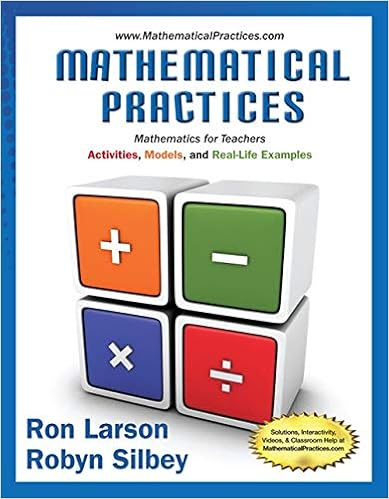# 4.01 Introduction to Triangles .pdf - Geometry Writing...

• 3

This preview shows page 1 out of 3 pages.

##### We have textbook solutions for you!
The document you are viewing contains questions related to this textbook.The document you are viewing contains questions related to this textbook.
Chapter 10 / Exercise 31
Mathematical Practices, Mathematics for Teachers: Activities, Models, and Real-Life Examples
LarsonExpert VerifiedUnformatted text preview: Geometry Writing Assignment – Introduction to Triangles Each problem is worth 5 points Total Points: 50 Solve the problems pertaining triangles. Be sure to show all work when needed. 1. Explain your reasoning. 2 RW = RU . Kendra disagrees. Is either of them correct? 3 side lengths are AB=18 AC=18 BC=27 6x+8x+4x=18x 63-54=9 18*3=54 x=3 triangle ABC is an isosceles triangle a. Based on the figure, Jordan says that b. If QW = 27 inches, find QT. Triangle ABC is an isosceles triangle because sides AB and AC are both the same length 2. Jordan says that from the information supplied in the diagram, she can conclude that R is on the perpendicular bisector of QS . Robert disagrees. Is either of them correct? Explain your reasoning. There is not enough information given to find x. To find the value of x there would need to be markings indicating whether the segments drawn from the ray are congruent to each other. 3. Franklin measured and labeled the angles of a triangle as shown. Jennifer says that at least one of his measures is incorrect. Explain in at least two different ways how Jennifer knows that this is true. Perpendicular bisectors, medians, and altitudes of a triangle all go to an opposite vertex they also pass through the midpoint. Medians and altitude start from 1 of the 3 vertex. Some differences are that perpendicular bisectors must be 90 degrees or it is a median. Altitude is the only term out of 3 that does not start from a vertex it has to start from the base. 4. Joey says that triangle XYZ is obtuse. Robin disagrees because there are more acute angles then there are obtuse angles therefore it must be acute. Is either one of them correct? Explain your reasoning. Joey is correct that XYZ is obtuse because angle Z is more than 90 degrees but less than 180 5. Compare and contrast the perpendicular bisectors and angle bisectors of a triangle. How are they alike? How are they different? Angle bisector cut an angle into 2 congruent angles but perpendicular bisectors are 2 lines the meet to form right angles at the midpoint line. They are alike because they both bisect something in a triangle and thy are both different because 1 cuts angles and another cuts line segments. 6. Compare and contrast the perpendicular bisectors, medians, and altitudes of a triangle. How are they alike? How are they different? Perpendicular bisectors, medians, and altitudes of a triangle all go to an opposite vertex they also pass through the midpoint. Medians and altitude start from 1 of the 3 vertex. Some differences are that perpendicular bisectors must be 90 degrees or it is a median. Altitude is the only term out of 3 that does not start from a vertex it has to start from the base. 7a. Classify triangle ABC by its sides if side AB = 6x, side AC = 4x + 6 and side BC = 8x + 3. The perimeter of triangle ABC is 63. Show all your work. side lengths are AB=18 AC=18 BC=27 6x+8x+4x=18x 63-54=9 18*3=54 x=3 triangle ABC is an isosceles triangle b. Explain your reasoning. Triangle ABC is an isosceles triangle because sides AB and AC are both the same length 8. Is there enough information given to find the value of X. Explain your reasoning. There is not enough information given to find x. To find the value of x there would need to be markings indicating whether the segments drawn from the ray are congruent to each other. ...
View Full Document

•••